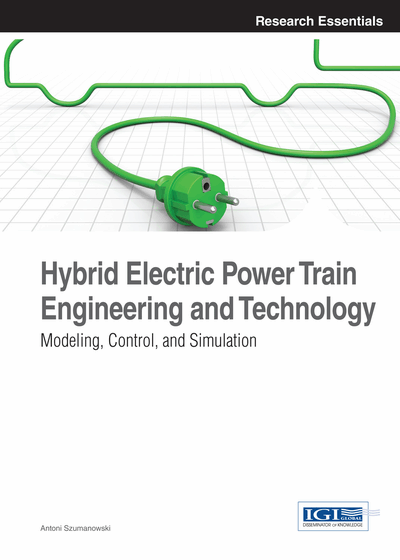# Generic Models of Electric Machine Applications in Hybrid Electric Vehicles Power Train Simulations

DOI: 10.4018/978-1-4666-4042-9.ch004

## Abstract

Chapter 4 presents an approach to obtain the power simulation model of electric machines that would be practically useful in hybrid power train simulation studies. The induction motor (AC) and the permanent magnet motor’s (PM) mathematical dynamic models are based on the necessary and fundamental knowledge conveyed in the previous chapter. These generic models are here adapted to the hybrid power train requirements, while the mechanical characteristics of the vehicle’s driving system are relegated to the background. The vector field oriented control of induction and permanent magnet motors is applied in the conducted mathematical modeling. The influence of the controlled voltage frequency is discussed as well. In the case of permanent magnet motors, the adjusted method of magnetic field weakening is very important during pulse modulation (PWM) control. The chapter presents the model of synchronous permanent motor magnetic field weakening. The basic simulation studies’ results dedicated especially to the above-mentioned electric motors are included. One of the targets of these simulations is the determination of these electric machines’ static characteristics (motor’s map) as the function: output mechanical torque versus the motors’ shaft rotational speed. This feature is indicated as the map of electric machines connected with its efficiency in a four quarterly operation (4Q), which means the operation of the motor/generator mode in two directions of the shaft rotational speed, which appears very useful in practice.
Chapter Preview
Top

## 1. Approach To A Power Simulation Model Of A Driving System With An Ac Induction Motor

### 1.1. Inverter/Motor Control Strategy

Before presenting the relationships between motor performance and motor design parameters, a description of the inverter/ motor control strategy is presented. This description is the fundamental basis for most thoughts involved in the selection and design of the motor for the particular application of the motor in the traction drive system.

The inverter/motor control strategy for maximum motor output is shown in Figure 1.

The motor torque-speed curve has the expected profile for a wide-speed range traction drive. Three regions of operation characterize this profile: constant torque, constant power and slip limited.

The constant torque region lies from a standstill point to the corner point speed (base speed). In this region, the inverter operates in the PWM mode to supply the adjustable voltage and frequency of the motor.

The alternative current AC voltage is adjusted as speed (frequency) changes to maintain constant flux density in the motor, as shown in Figure 1. The alternative current voltage, therefore, basically increases proportionally with speed (frequency). The frequency of the voltage inducted in the rotor (sleep frequency) is held constant and the AC current is nearly constant. This produces a nearly constant torque. Of course, the output power of the motor is proportional to speed, so power increases linearly, with speed up to the corner point speed. This method of control is possible until the AC voltage reaches the maximum available from the inverter. This juncture is the corner point and is the end of the constant torque region and the beginning of the constant power region. In this case, the behavior of the alternative current AC motor is the same as a separately excited, direct current DC motor.

The constant power region lies from the corner point speed to maximum speed (speed at the end of the constant power region).

In this region, the inverter supplies adjustable frequency to the motor. The alternative current AC voltage is no longer adjustable, as the inverter is producing the maximum voltage, so AC voltage is constant. The results in the flux density decrease as the reciprocal of speed (frequency). The slip frequency is increased and alternative current voltage (AC) is nearly constant. This produces a torque, which decreases as the reciprocal of speed, while power out of the motor is nearly constant. This method of control is possible until the motor reaches the breakdown at its slip limit. The important juncture is the maximum speed or the end of the constant power region and the beginning of the slip limited region, which lies behind the constant power region. Any further increases in speed must be carried out at a constant slip frequency, with alternative (AC) current decreases. The voltage and the flux density behave as in the constant power region. This results in the torque, which decreases as the reciprocal of speed squared, and a resulting decrease in power.

### 1.2. Effect of Transmission

The torque can be transmitted to the wheels via the gearbox with two or more speed transmissions. In time, the gears shift from a low gear (high transmission ratio) to a high gear (low transmission ratio), and the motor will operate more easily in the constant power range. The drive in the city traffic cycle up to a maximum speed, for example 70 km/h (or more), is only possible using one basic gear step ratio. The best solution is permanent use of CVT (Continuously Variable Transmission) between the motor and traction wheels. This way is costly, but it offers the possibility of minimizing the motor copper losses, and better adjusts the motor parameters to vehicle driving requirements.

## Complete Chapter List

Search this Book:
Reset